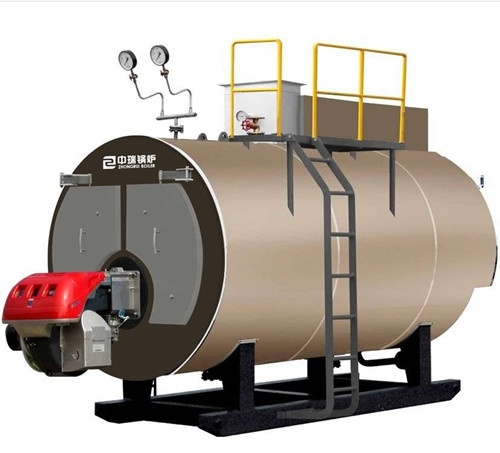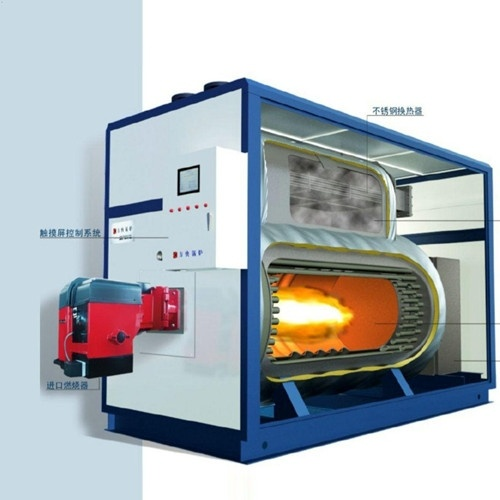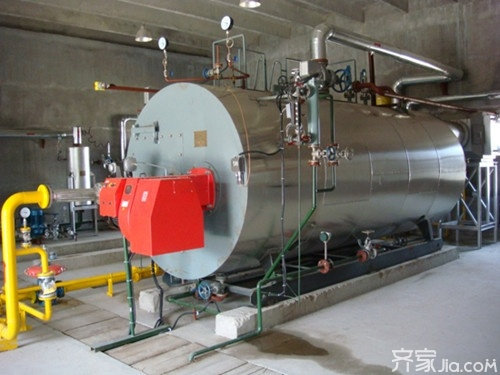|

# 热水锅炉价格 热水锅炉发出奇怪响声怎么办

热水锅炉就是生产热水的锅炉，是指利用燃料燃烧释放的热能或其它的热能（如电能、太阳能等）把水加热到额定温度的一种热能设备。一般我们比较常见的就是电热水锅炉和燃煤热水锅炉。热水锅炉主要有采暖和洗浴两种用途。高中、大学应该都会有，包括北方的澡堂，也是有很多人在使用热水锅炉。对于有这方面需求的朋友们来说，热水锅炉价格是他们关注的焦点。下面就让我来为大家详细介绍热水锅炉价格，供大家选购时参考。热水锅炉介绍

热水锅炉包括电热水锅炉、燃油热水锅炉、燃气热水锅炉及燃煤热水锅炉等，热水锅炉就是生产热水的锅炉，是指利用燃料燃烧释放的热能或其它的热能（如电能、太阳能等）把水加热到额定温度的一种热能设备。《特种设备安全监察条例》所定义的锅炉是指利用各种燃料、电或者其他能源，将所盛装的液体加热到一定的参数，并对外输出热能的设备。其范围规定为容积大于或者等于30L的承压蒸汽锅炉；出口水压大于或者等于0.1MPa（表压)，且额定功率大于或者等于0.1Mw的承压热水锅炉；有机热载体锅炉。摘自中华人民共和国《特种设备安全监察条例》

热水锅炉优缺点

常压锅炉也称无压热水锅炉，是指锅炉顶部通大气，不承受供热系统的水柱静压力。也就是相当一个”开式热水箱“。循环水泵只能装在热水供水干管上，而且要装止回阀和流量调节阀，所以循环水泵并不起使水循环的作用，而起加压水的作用，实际是加压水泵，这就像给水工程中的冷水加压泵一样．把蓄水池的水抽送到水塔，再靠重力送到用户。

优点：

(1)永无爆炸危险，可不进行监检。

(2)不必考虑锅炉房的泄压问题．

(3)节省钢材与简化工艺．

(4)报废锅炉再用。

(5)取消补水泵。

(6)停电保护好。

(7)经久耐用．

(8)节省用户各项投资

缺点：

(1)系统循环能耗问题。

(2)循环水泵人口静压力较低的问题。

(3)循环水泵入口水温较高的问题。

(4)锅水与大气直接接触的问题。热水锅炉适用范围

热水锅炉广泛用于酒店、学校、宾馆、小区等企事业单位的采暖、洗浴和生活热水等。

热水锅炉价格

遂昌大型温泉改造生物质热水锅炉售价50000元，不过送到家光运费就要600多元了哦。

22吨承压热水锅炉/法罗力燃气燃油钢制工业锅炉/prex3GN-CN售价50000元。一吨的话就是100000元，运费1000元。

1.5吨承压热水锅炉/法罗力燃气燃油钢制工业锅炉/宝力售价50000元。

2吨常压热水锅炉/法罗力燃气燃油钢制工业锅炉/宝力power售价50000元。

能率牌燃气锅炉节能燃气锅炉供暖节能热水设备燃气热水锅炉售价50000元。

供应常压燃油燃气热水炉常压电热水炉生物质热水锅炉蒸汽开水售价50000元。

Dupow电热水锅炉储水式立式大容量商用大型电热水器泡澡洗浴售价4180元。

家用燃煤暖气锅炉/养殖取暖炉/采暖地暖热水锅炉数控节能自动型售价1290元。家用燃煤采暖锅炉/反烧节能锅炉/采暖炉/土暖气地暖热水锅炉包邮售价1380元。

美国佳福斯电采暖炉家用采暖洗浴两用恒温即热式电热水器电热锅炉售价8088元。

茶水炉燃煤烧柴烧热水锅炉建筑工地专用茶水锅炉生活用茶水炉售价650元。

家用采暖节能小型常压热水蒸汽锅炉做豆腐杀灭菌蒸酒锅炉燃煤木柴售价900元。

武汉洲洋供暖进口德国威能壁挂炉标准型24kw燃气采暖/热水锅炉售价10800元。

板式换热器锅炉配件热水锅炉浴池专用锅炉热水交换器售价320元。

注：此价格仅供参考！由于地域不同，当然价格也会有所差异。如需了解更多相关价格详情，请以当地经销商提供为准！

编辑总结：锅炉有家用和商用的，有沐浴和取暖两种用途，也有既可以沐浴和取暖两种共用的，也有一些进口的比较贵，现在市场上充斥着各种各样的热水器。热水锅炉已经不是很多人在用了。

`声明：本文由入驻焦点开放平台的作者撰写，除焦点官方账号外，观点仅代表作者本人，不代表焦点立场错误信息举报电话： 400-099-0099，邮箱：jubao@vip.sohu.com，或点此进行意见反馈，或点此进行举报投诉。`A B C D E F G H J K L M N P Q R S T W X Y Z
A - B - C - D - E
• A
• 鞍山
• 安庆
• 安阳
• 安顺
• 安康
• 澳门
• B
• 北京
• 保定
• 包头
• 巴彦淖尔
• 本溪
• 蚌埠
• 亳州
• 滨州
• 北海
• 百色
• 巴中
• 毕节
• 保山
• 宝鸡
• 白银
• 巴州
• C
• 承德
• 沧州
• 长治
• 赤峰
• 朝阳
• 长春
• 常州
• 滁州
• 池州
• 长沙
• 常德
• 郴州
• 潮州
• 崇左
• 重庆
• 成都
• 楚雄
• 昌都
• 慈溪
• 常熟
• D
• 大同
• 大连
• 丹东
• 大庆
• 东营
• 德州
• 东莞
• 德阳
• 达州
• 大理
• 德宏
• 定西
• 儋州
• 东平
• E
• 鄂尔多斯
• 鄂州
• 恩施
F - G - H - I - J
• F
• 抚顺
• 阜新
• 阜阳
• 福州
• 抚州
• 佛山
• 防城港
• G
• 赣州
• 广州
• 桂林
• 贵港
• 广元
• 广安
• 贵阳
• 固原
• H
• 邯郸
• 衡水
• 呼和浩特
• 呼伦贝尔
• 葫芦岛
• 哈尔滨
• 黑河
• 淮安
• 杭州
• 湖州
• 合肥
• 淮南
• 淮北
• 黄山
• 菏泽
• 鹤壁
• 黄石
• 黄冈
• 衡阳
• 怀化
• 惠州
• 河源
• 贺州
• 河池
• 海口
• 红河
• 汉中
• 海东
• 怀来
• I
• J
• 晋中
• 锦州
• 吉林
• 鸡西
• 佳木斯
• 嘉兴
• 金华
• 景德镇
• 九江
• 吉安
• 济南
• 济宁
• 焦作
• 荆门
• 荆州
• 江门
• 揭阳
• 金昌
• 酒泉
• 嘉峪关
K - L - M - N - P
• K
• 开封
• 昆明
• 昆山
• L
• 廊坊
• 临汾
• 辽阳
• 连云港
• 丽水
• 六安
• 龙岩
• 莱芜
• 临沂
• 聊城
• 洛阳
• 漯河
• 娄底
• 柳州
• 来宾
• 泸州
• 乐山
• 六盘水
• 丽江
• 临沧
• 拉萨
• 林芝
• 兰州
• 陇南
• M
• 牡丹江
• 马鞍山
• 茂名
• 梅州
• 绵阳
• 眉山
• N
• 南京
• 南通
• 宁波
• 南平
• 宁德
• 南昌
• 南阳
• 南宁
• 内江
• 南充
• P
• 盘锦
• 莆田
• 平顶山
• 濮阳
• 攀枝花
• 普洱
• 平凉
Q - R - S - T - W
• Q
• 秦皇岛
• 齐齐哈尔
• 衢州
• 泉州
• 青岛
• 清远
• 钦州
• 黔南
• 曲靖
• 庆阳
• R
• 日照
• 日喀则
• S
• 石家庄
• 沈阳
• 双鸭山
• 绥化
• 上海
• 苏州
• 宿迁
• 绍兴
• 宿州
• 三明
• 上饶
• 三门峡
• 商丘
• 十堰
• 随州
• 邵阳
• 韶关
• 深圳
• 汕头
• 汕尾
• 三亚
• 三沙
• 遂宁
• 山南
• 商洛
• 石嘴山
• T
• 天津
• 唐山
• 太原
• 通辽
• 铁岭
• 泰州
• 台州
• 铜陵
• 泰安
• 铜仁
• 铜川
• 天水
• 天门
• W
• 乌海
• 乌兰察布
• 无锡
• 温州
• 芜湖
• 潍坊
• 威海
• 武汉
• 梧州
• 渭南
• 武威
• 吴忠
• 乌鲁木齐
X - Y - Z
• X
• 邢台
• 徐州
• 宣城
• 厦门
• 新乡
• 许昌
• 信阳
• 襄阳
• 孝感
• 咸宁
• 湘潭
• 湘西
• 西双版纳
• 西安
• 咸阳
• 西宁
• 仙桃
• 西昌
• Y
• 运城
• 营口
• 盐城
• 扬州
• 鹰潭
• 宜春
• 烟台
• 宜昌
• 岳阳
• 益阳
• 永州
• 阳江
• 云浮
• 玉林
• 宜宾
• 雅安
• 玉溪
• 延安
• 榆林
• 银川
• Z
• 张家口
• 镇江
• 舟山
• 漳州
• 淄博
• 枣庄
• 郑州
• 周口
• 驻马店
• 株洲
• 张家界
• 珠海
• 湛江
• 肇庆
• 中山
• 自贡
• 资阳
• 遵义
• 昭通
• 张掖
• 中卫

1室1厅1厨1卫1阳台

1
2
3
4
5

0
1
2

1

1

0
1
2
3报名成功，资料已提交审核A B C D E F G H J K L M N P Q R S T W X Y Z
A - B - C - D - E
• A
• 鞍山
• 安庆
• 安阳
• 安顺
• 安康
• 澳门
• B
• 北京
• 保定
• 包头
• 巴彦淖尔
• 本溪
• 蚌埠
• 亳州
• 滨州
• 北海
• 百色
• 巴中
• 毕节
• 保山
• 宝鸡
• 白银
• 巴州
• C
• 承德
• 沧州
• 长治
• 赤峰
• 朝阳
• 长春
• 常州
• 滁州
• 池州
• 长沙
• 常德
• 郴州
• 潮州
• 崇左
• 重庆
• 成都
• 楚雄
• 昌都
• 慈溪
• 常熟
• D
• 大同
• 大连
• 丹东
• 大庆
• 东营
• 德州
• 东莞
• 德阳
• 达州
• 大理
• 德宏
• 定西
• 儋州
• 东平
• E
• 鄂尔多斯
• 鄂州
• 恩施
F - G - H - I - J
• F
• 抚顺
• 阜新
• 阜阳
• 福州
• 抚州
• 佛山
• 防城港
• G
• 赣州
• 广州
• 桂林
• 贵港
• 广元
• 广安
• 贵阳
• 固原
• H
• 邯郸
• 衡水
• 呼和浩特
• 呼伦贝尔
• 葫芦岛
• 哈尔滨
• 黑河
• 淮安
• 杭州
• 湖州
• 合肥
• 淮南
• 淮北
• 黄山
• 菏泽
• 鹤壁
• 黄石
• 黄冈
• 衡阳
• 怀化
• 惠州
• 河源
• 贺州
• 河池
• 海口
• 红河
• 汉中
• 海东
• 怀来
• I
• J
• 晋中
• 锦州
• 吉林
• 鸡西
• 佳木斯
• 嘉兴
• 金华
• 景德镇
• 九江
• 吉安
• 济南
• 济宁
• 焦作
• 荆门
• 荆州
• 江门
• 揭阳
• 金昌
• 酒泉
• 嘉峪关
K - L - M - N - P
• K
• 开封
• 昆明
• 昆山
• L
• 廊坊
• 临汾
• 辽阳
• 连云港
• 丽水
• 六安
• 龙岩
• 莱芜
• 临沂
• 聊城
• 洛阳
• 漯河
• 娄底
• 柳州
• 来宾
• 泸州
• 乐山
• 六盘水
• 丽江
• 临沧
• 拉萨
• 林芝
• 兰州
• 陇南
• M
• 牡丹江
• 马鞍山
• 茂名
• 梅州
• 绵阳
• 眉山
• N
• 南京
• 南通
• 宁波
• 南平
• 宁德
• 南昌
• 南阳
• 南宁
• 内江
• 南充
• P
• 盘锦
• 莆田
• 平顶山
• 濮阳
• 攀枝花
• 普洱
• 平凉
Q - R - S - T - W
• Q
• 秦皇岛
• 齐齐哈尔
• 衢州
• 泉州
• 青岛
• 清远
• 钦州
• 黔南
• 曲靖
• 庆阳
• R
• 日照
• 日喀则
• S
• 石家庄
• 沈阳
• 双鸭山
• 绥化
• 上海
• 苏州
• 宿迁
• 绍兴
• 宿州
• 三明
• 上饶
• 三门峡
• 商丘
• 十堰
• 随州
• 邵阳
• 韶关
• 深圳
• 汕头
• 汕尾
• 三亚
• 三沙
• 遂宁
• 山南
• 商洛
• 石嘴山
• T
• 天津
• 唐山
• 太原
• 通辽
• 铁岭
• 泰州
• 台州
• 铜陵
• 泰安
• 铜仁
• 铜川
• 天水
• 天门
• W
• 乌海
• 乌兰察布
• 无锡
• 温州
• 芜湖
• 潍坊
• 威海
• 武汉
• 梧州
• 渭南
• 武威
• 吴忠
• 乌鲁木齐
X - Y - Z
• X
• 邢台
• 徐州
• 宣城
• 厦门
• 新乡
• 许昌
• 信阳
• 襄阳
• 孝感
• 咸宁
• 湘潭
• 湘西
• 西双版纳
• 西安
• 咸阳
• 西宁
• 仙桃
• 西昌
• Y
• 运城
• 营口
• 盐城
• 扬州
• 鹰潭
• 宜春
• 烟台
• 宜昌
• 岳阳
• 益阳
• 永州
• 阳江
• 云浮
• 玉林
• 宜宾
• 雅安
• 玉溪
• 延安
• 榆林
• 银川
• Z
• 张家口
• 镇江
• 舟山
• 漳州
• 淄博
• 枣庄
• 郑州
• 周口
• 驻马店
• 株洲
• 张家界
• 珠海
• 湛江
• 肇庆
• 中山
• 自贡
• 资阳
• 遵义
• 昭通
• 张掖
• 中卫• 手机• 分享
• 设计
免费设计
• 计算器
装修计算器
• 入驻
合作入驻
• 联系
联系我们
• 置顶
返回顶部